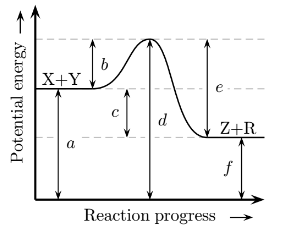# Problem: For the reaction X + Y → Z + R which arrow represents the activation energy of the forward reaction? 1. e 2. f 3. a 4. c 5. d 6. b

###### FREE Expert Solution
93% (374 ratings)
###### Problem Details

For the reaction

X + Y → Z + R

which arrow represents the activation energy of the forward reaction?

1. e

2. f

3. a

4. c

5. d

6. bWhat scientific concept do you need to know in order to solve this problem?

Our tutors have indicated that to solve this problem you will need to apply the Energy Diagram concept. If you need more Energy Diagram practice, you can also practice Energy Diagram practice problems.

What is the difficulty of this problem?

Our tutors rated the difficulty ofFor the reaction X + Y → Z + R which arrow represents the ...as medium difficulty.

How long does this problem take to solve?

Our expert Chemistry tutor, Sabrina took 1 minute and 34 seconds to solve this problem. You can follow their steps in the video explanation above.

What professor is this problem relevant for?

Based on our data, we think this problem is relevant for Professor Carroll's class at TN TECH.